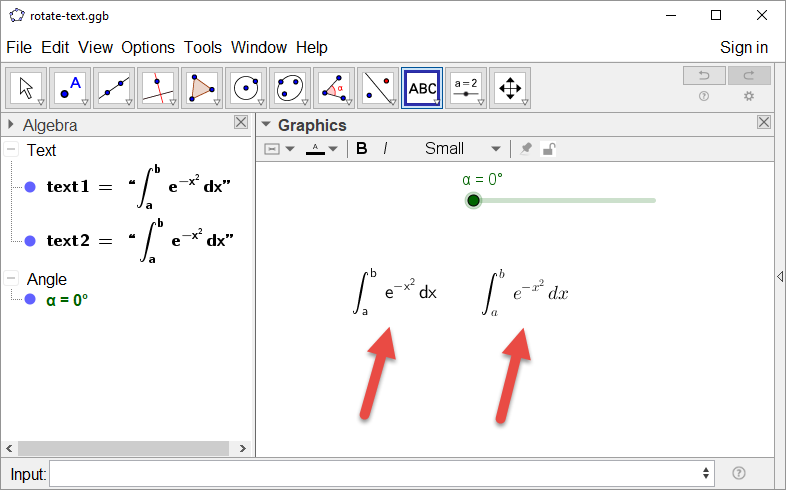# Bug in RotateText()?

hjbortol shared this problem 5 years ago
Solved

Hi!

I'm trying to use RotateText() with a math expression. The text is displayed correctly at the Algebra Window but, in the View Windows, the LaTeX command \rotatebox{} appears. Is this a bug?

Another question: why, in text1, the math formula is not displayed using the usual LaTeX fonts?

Thanks, Humberto.11

RotateText(FormulaText("\int_{a}^{b} e^{-x^2} \, dx"), α)

(RotateText prepends rotatebox{ and appends } to your text so if you use \$ it will have some unwanted switches between textmode and math mode)1

Here it is!1

Zbynek, your suggestion worked! However, the rendered text is not typeset in Computer Modern fonts as usual math text does. Is there a way to make RotateText + FormulaText render math text using Computer Modern fonts? Thanks!2

intenta

RotateText(FormulaText("\int_{a}^{b} e^{-x^2} \, dx"), α)

y

text2 = "\int_{a}^{b}{ e^{-x^2}}" as Latex

then RotateText(text2, α)

saludos

Files: foro.ggb